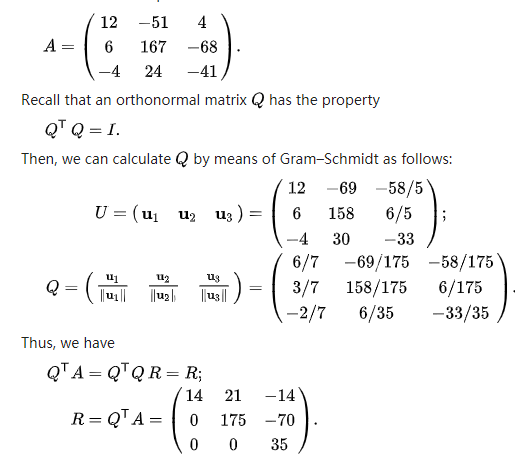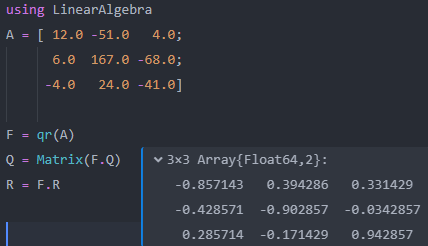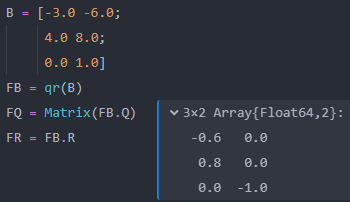# QR分解，LinearAlgebra包中计算结果疑问，望指教！using LinearAlgebra
A = [ 12.0 -51.0   4.0;
6.0  167.0 -68.0;
-4.0   24.0 -41.0]

F = qr(A)
Q = Matrix(F.Q)
R = F.RB = [-3.0 -6.0;
4.0 8.0;
0.0 1.0]
FB = qr(B)
FQ = Matrix(FB.Q)
FR = FB.R


wiki上的算法适合手算，不一定是最有效率的算法。

Let {\displaystyle \mathbf {x} }be an arbitrary real m -dimensional column vector of {\displaystyle A}such that {\displaystyle |\mathbf {x} |=|\alpha |}for a scalar α . If the algorithm is implemented using floating-point arithmetic, then α should get the opposite sign as the k -th coordinate of {\displaystyle \mathbf {x} }, where {\displaystyle x_{k}}is to be the pivot coordinate after which all entries are 0 in matrix A 's final upper triangular form, to avoid loss of significance.

https://en.wikipedia.org/wiki/QR_decomposition#Using_Householder_reflections

QR函数API中有如下解释：
qr(A, pivot=Val(false); blocksize) -> F

The returned object F stores the factorization in a packed format:

• if pivot == Val(true) then F is a QRPivoted object,
• otherwise if the element type of A is a BLAS type (Float32, Float64, ComplexF32 or ComplexF64), then F is a QRCompactWY object,
• otherwise F is a QR object.

qr(A, Val(true))

1 个赞

julia> A
3×2 Array{Int64,2}:
1  -4
2   3
2   2

julia> F=qr(A)
LinearAlgebra.QRCompactWY{Float64,Array{Float64,2}}
Q factor:
3×3 LinearAlgebra.QRCompactWYQ{Float64,Array{Float64,2}}:
-0.333333   0.933333  -0.133333
-0.666667  -0.333333  -0.666667
-0.666667  -0.133333   0.733333
R factor:
2×2 Array{Float64,2}:
-3.0  -2.0
0.0  -5.0

julia> F.Q
3×3 LinearAlgebra.QRCompactWYQ{Float64,Array{Float64,2}}:
-0.333333   0.933333  -0.133333
-0.666667  -0.333333  -0.666667
-0.666667  -0.133333   0.733333

julia> Matrix(F.Q)
3×2 Array{Float64,2}:
-0.333333   0.933333
-0.666667  -0.333333
-0.666667  -0.133333


F.Q无法进行求逆运算，如果强制转换类型为Matrix，则矩阵尺寸少了一列，这是什么原因造成的？这就相当于只能看到QR分解的结果，但是却不能进一步使用

julia> A = rand(3, 2);

julia> F = qr(A);

julia> F.Q * Matrix(I, 3, 3)
3×3 Array{Float64,2}:
-0.543092   0.776157   0.320361
-0.741833  -0.264778  -0.616097
-0.393364  -0.572252   0.719578

1 个赞